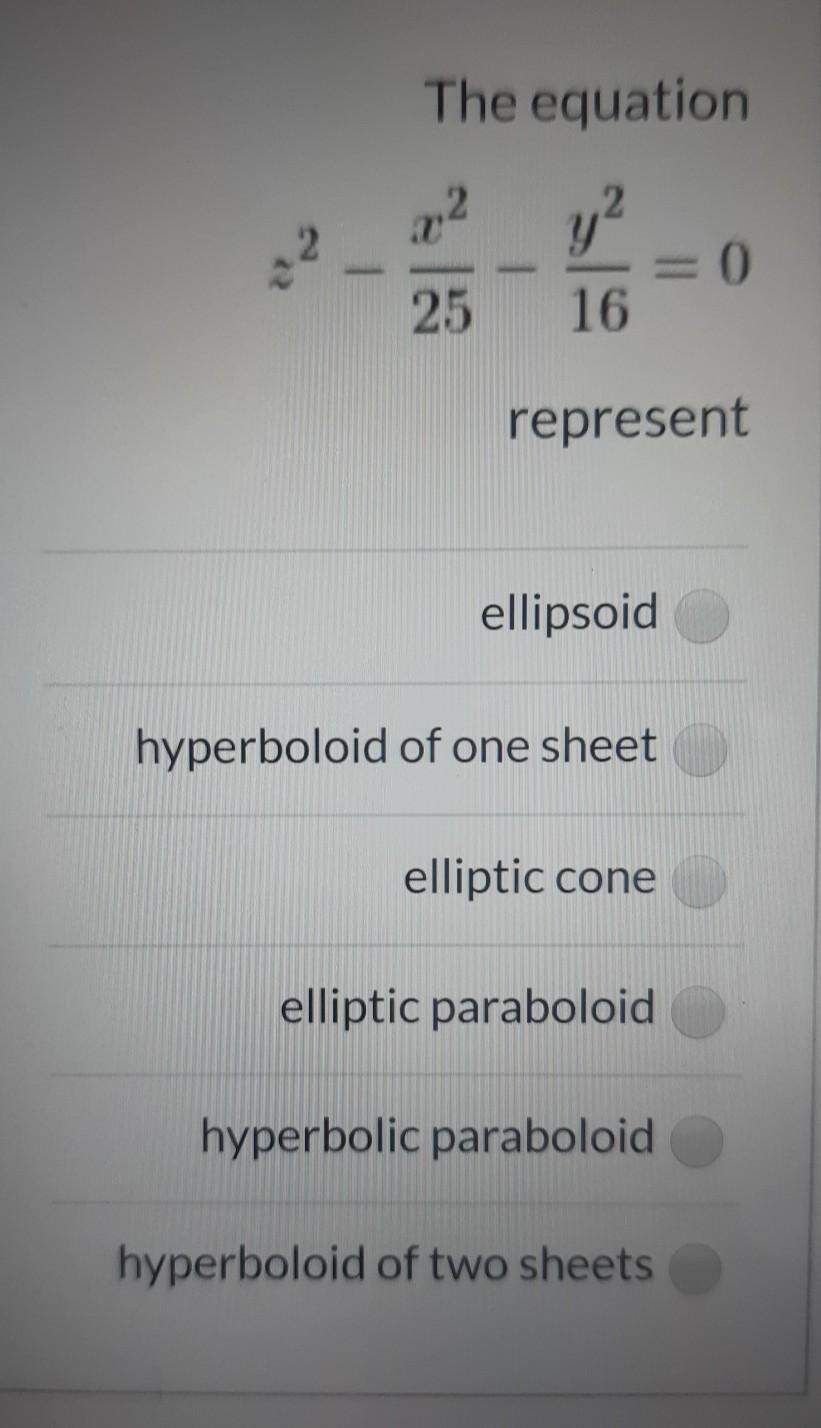### Create an Account

Home / Questions / The equation x2 y? 16 = 0 25 represent ellipsoid hyperboloid of one sheet elliptic cone el...

# The equation x2 y? 16 = 0 25 represent ellipsoid hyperboloid of one sheet elliptic cone elliptic paraboloid hyperbolic paraboloid hyperboloid of two sheets

The equation x2 y? 16 = 0 25 represent ellipsoid hyperboloid of one sheet elliptic cone elliptic paraboloid hyperbolic paraboloid hyperboloid of two sheetsMay 08 2021 View more View LessSubscribe To Get Solution